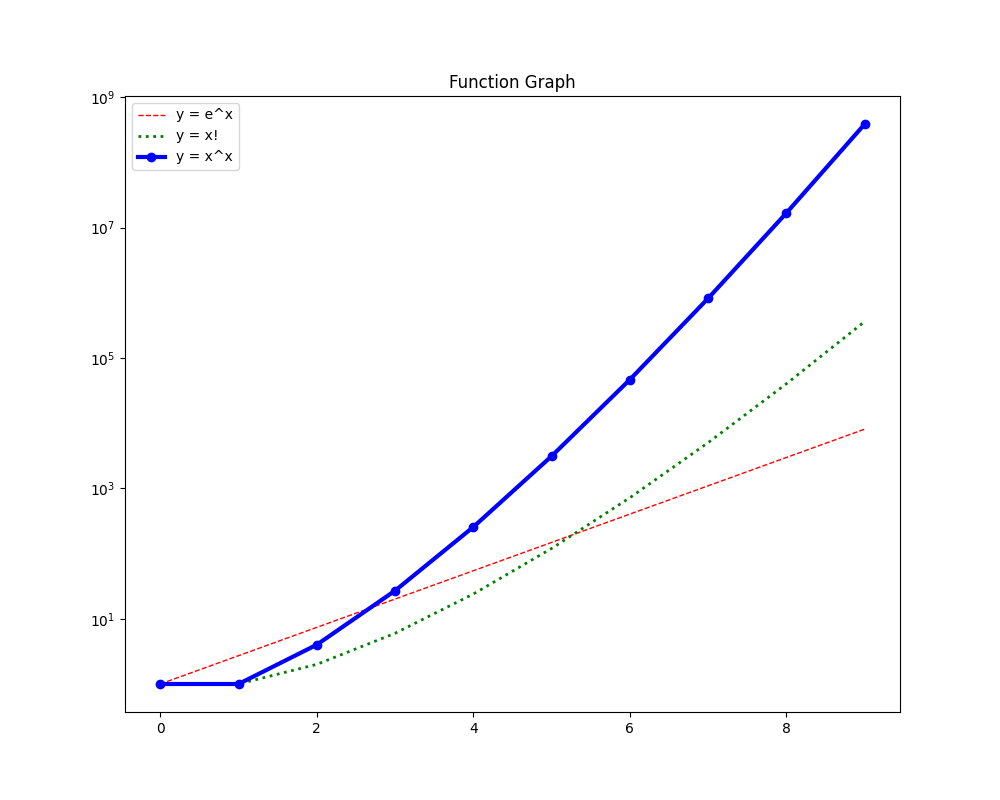By Long Luo# 对数放缩法 (Zoom)

$A = \log_{2}{(2^{100!})} = 100!$

$B = \log_{2}{2^{100}!}= \log_{2}{2^{100}} + \log_{2}{(2^{100} - 1)} + \dots + 1 + 0$

$B < 100 \cdot 2^{100} < 128 \cdot 2^{100} = 2^7 \cdot 2^{100} = 2^{107}$

$100!$ 至少有 $(100 - 64 + 1) = 37$ 项是大于等于 $64 = 2^6$ ，也就是 $(2^6)^{37}=2^{222}$

# 斯特林公式 (Stirling’s Approximation)

$n! \sim \sqrt{2 \pi n}(\frac{n}{e})^n = \sqrt{2 \pi} n^{\frac{n + 1}{2}}e^{-n}$

$\ln{n!} \sim \frac{1}{2} ln(2 \pi) + (n+\frac{1}{2}) ln(n) - n$

$\ln(n!) \sim n(ln(n) - 1)$

$\begin{array}{l} A = ln(2^{100!}) = 100! ln(2) \\ B = ln(2^{100}!) \sim 2^{100}(ln(2^{100}) - 1) = 2^{100}(100 ln(2) - 1) \end{array}$

$2^{100!} \gg 2^{100}!$

# 数列法

$F_n= \frac{2^{n!}}{(2^n)!}$

$n = 1$ 时，

$F_1 = \frac{2^{1!}}{(2^1)!} = \frac{2^{1}}{(2)!} = \frac{2}{2} = 1$

$n = k + 1$ 时，

$F_{k + 1} = \frac{2^{(k + 1)!}}{2^{k + 1}!} = F_k \cdot \frac{2^{(k + 1)! - k!}}{2^{k + 1} \cdot (2^{k + 1} - 1) \cdots (2^k + 1)}$

1. $(n + 1)! - n! < n2^n$ , $A_{n+1} < A_n$
2. $(n + 1)! - n! > (n + 1)2^n$ , $A_{n+1} > A_n$

$\begin{array}{l} F_2 = 0.25 \\ F_3 = 0.0015\cdots \\ F_4 = 8.018\cdots \times 10^{−7} \\ F_5 = 5.05\cdots \\ F_6 = 4.34\cdots \times 10^{127} \\ F_7 = 4.02\cdots \times 10^{1301} \\ \end{array}$

$2^{100!}$$(2^{100})!$超出想象的大。

# 简洁法

$n! = 1 \times 2 \times \cdots n$ ，而 $n^n = n \times n \times \cdots n$

$n > 2$ , $n^n \gg n!$

$B = (2^{100})! < (2^{100})^{2^{100}} = 2^{100 \cdot 2^{100}}$

$B = (2^{100})! < 2^{2^{107}}$

$\begin{array}{l} 100! = 2^{50} \cdot 2^{25} \cdot 2^{12} \cdot 2^6 \cdot 2^3 \cdot 2 \cdot 3^{32} \cdots 5^{20} \cdots 11 \cdots 97 \\ = 2^{97} \cdot 11 \cdot 13 \cdots 97 \\ \gg 2^{10} \cdot 2^{97} = 2^{107} \\ \end{array}$